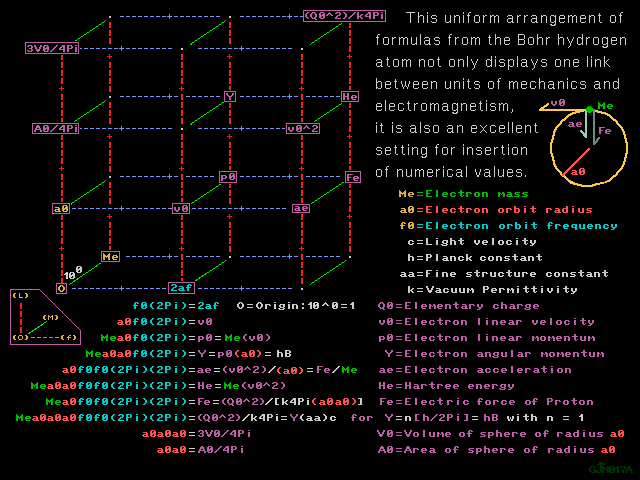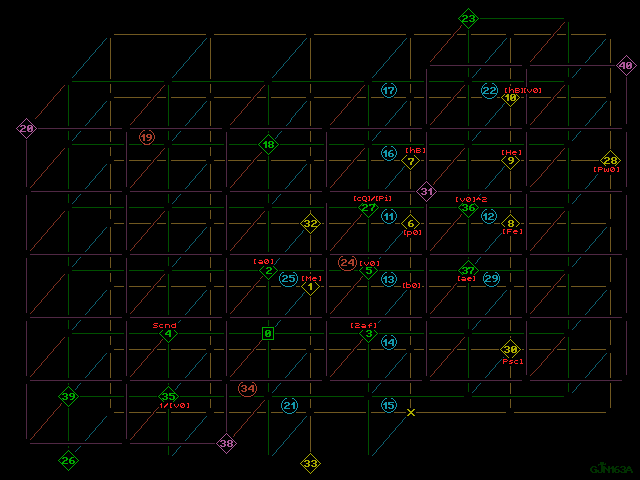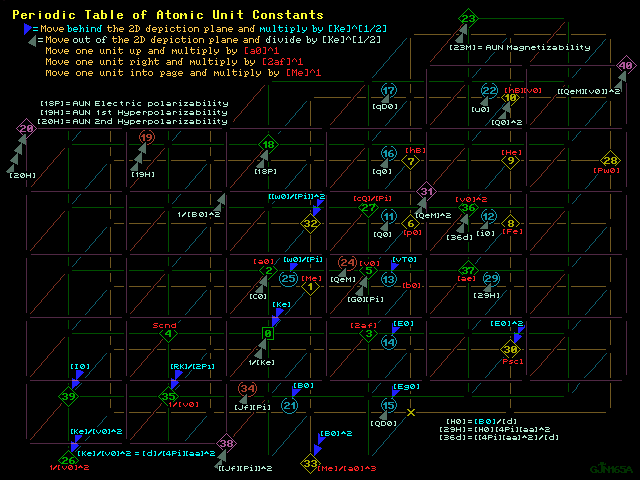```Color print  Values table    1280 X 960 GIF    B & W print

Tutorial back, Symbol key     Planck constants map, Tutorial next

Wedges as displacement into the fourth dimensionColor print      1280 X 960 GIF      Grayscale printColor print      1280 X 960 GIF      B & W printColor print      1280 X 960 GIF      B & W print

The graphic just above is a 2D portrayal of a 3D map, but includes
representation of displacement into 4D, where multiplication by a
factor of 1/{([4Pi][k])^[1/2]} = ([Ke]^[1/2]) is symbolized by a
single wedge expanding away from view and behind the 2D depiction
plane, and division by that factor is signified by a single wedge
expanding toward being in front of the depiction plane of the page:

Moving two wedges toward behind the depiction plane of this image is
multiplication by the Coulomb constant [Ke] in (Meters per Farad)^+1
and moving two wedges toward being in front of the image is division
by the Coulomb constant [Ke] in (Meters per Farad)^+1 .

Numbers between asterisks below refer to locations in the graphics
above: four different projections of that vector space populated by
single occurrences or combinations of pre-existing, pre-defined and
familiar atomic unit constants whose current CODATA 2014 values mesh
to form a self-validating, internally consistent and coherent space:
every vector from the origin to a principal plot point and every
vector between principal plot points is recognizable as a single
constant or some combination of constants multiplied by no numerical
factor except one.  With this precedent established for both the
validity of the mapping technique and the state of coherence, it can
only be called compelling evidence that only one numerical value for
the Newtonian gravitational constant produces a state of coherence
in the periodic table of Planck unit constants.

GUNTERTABLE2 only print

---------------------------------++---------------------------------
GUNTERTABLE2 : for the Periodic Table of Atomic Unit Constants
---------------------------------++---------------------------------
POWER OF TEN | COMMENTS, NOTES                                 UNITS
---------------------------------++---------------------------------
AUN = Atomic unit of                          PUN = Planck unit of

0.0000000  1 = ^0 = [Anything]^0
*0*  = {^0}{[Me]^0}{[a0]^0}{[2af]^0}{{[Ke]^0}             ORIGIN

+  9.9536414  [Ke] = [hB][v0]/{[Q0]^2} = Coulomb constant   fradMetr
= [d]{[v0]^2}/([4Pi]{[aa]^2}) = [z0]{[v0]^2}/([c][4Pi]{[aa]^2})
= [v0][RK]/[2Pi] = [c^2][d]/[4Pi] = [a0][B0]/{[Me][Jf][Pi]}
*0*  = [Ke] = 1/{[4Pi][k]}                    = 1/[AUN permittivity]

- 30.0405110  [Me] = [PM][PL]/{[aa][a0]}           Kgrm=h2tzmetrNwtn
= 2[Rc][h]/([c]{[aa]^2}) = [Fe]/{[v0][2af]}        CuulhrtzTsla
= {[B0]^2}[AUN electric polarizability]            C2ulHnrym2tr
= {[Q0]^2}[d]/([a0][4Pi]{[aa]^2})              C2uljuulM2trT2la
= {[i0]^2}[RK]/([2af]{[v0]^2}[2Pi])            A2prh3tzm2trOhmm
*1*  = [Me]        = Electron mass = Natural unit of mass = AUN mass

- 10.2763989  [a0] =   {[Pi][B0][Jf]}^-.5 =  [aa]/{[Rc][4Pi]}   Metr
= [Re]/{[aa]^2} = {[Pi][B0]/[w0]}^-.5 = [Cw]/{[aa][2Pi]}
= [4Pi][k]{[hB]^2}/([Me]{[Q0]^2}) = [PM][PL]/{[aa][Me]}
*2*  = [a0] = {[hB]^2}/([Ke][Me]{[Q0]^2}) = Bohr radius = AUN length

- 20.2300403  [C0] = [a0]/[Ke] = [Q0]/[vT0]            Cuulvltt=Frad
= [G0][Pi]/[2af] = {[Q0]^2}/[He]              hrtzohmm=C2uljuul
*2*  = [a0]{[Ke]^-1}                               = AUN capacitance

+ 16.6163849  [2af] = [2Pi][f0] = [He]/[hB]            Hrtz=fradohmm
= {[2Pi]/[RK]}{[Ke]/[a0]} = [i0]/[Q0] = [QeM][B0]  CuulkgrmTsla
*3*  = [2af] = [Me]{[Q0]^4}{[Ke]^2}/{[hB]^3}         = AUN frequency

- 16.6163849  {[2Pi][f0]}^-1 = [hB]/[He] = [Q0]/[i0]   hrtz=FradOhmm
= {[RK]/[2Pi]}{[a0]/[Ke]} = {[QeM][B0]}^-1         cuulKgrmTsla
*4*  = [2af]^-1 = {[hB]^3}/([Me]{[Q0]^4}{[Ke]^2})         = AUN time

-  3.6136553  [G0][Pi] = [2Pi]/[RK] = [4Pi][aa]/[z0]       Smns=ohmm
= [4Pi][H0]{[aa]^2}/[E0] = [29H]/[E0]         A2prwaat=Amprvltt
*5*  = [a0][2af]{[Ke]^-1} = [v0]/[Ke]    = [Conductance quantum][Pi]

+  6.3399860  [v0] = [a0][He]/[hB] = {[Q0]^2}[Ke]/[hB]      HrtzMetr
= [Ke][2Pi]/[RK] = [G0][Ke][Pi] = [E0]/[B0]    CuulfradMetrwebr
*5*  = [a0][2af] = [a0]{2[Pi][f0]} = [Jf][Q0][Ke][Pi] = AUN velocity

- 23.7005250  [p0] = [hB]/[a0] = [Me][v0]               HrtzKgrmMetr
*6*  = [Me][a0][2af] = [Me][aa][c]                    = AUN momentum

- 33.9769238  [hB] = [h]/[2Pi] = [PM][PL][c]   hrtzJuul=HrtzKgrmM2tr
= {[Q0]^2}/{[4Pi][k][v0]} = [B0][Q0]{[a0]^2}       CuulM2trTsla
= {[Q0]^2}[Ke]/[v0] = [Q0][w0]/[Pi] = [He]/{[4Pi][Rc][c]}
= {[Q0]^2}[RK]/[2Pi] = [u0][Me]/[Q0] = [G]{[PM]^2}/[c]
*7*  = {[Q0]^2}[v0][d]/([4Pi]{[aa]^2}) = {[PQ]^2}[z0]   = AUN action
@7@  = [Me]{[a0]^2}[2af] = [PM]{[PL]^2}[Pf] = Natural unit of action

-  7.0841401  [Fe] =[Ke]{[Q0]^2}/{[a0]^2}              Juulmetr=Nwtn
=                  {[i0]^2}/({[v0]^2}[4Pi][k])     A2prHnrymetr
=              [RK]{[i0]^2}/{[aa][c][2Pi]}     A2prhrtzmetrOhmm
=         4{[aa]^2}{[w0]^2}/({[a0]^2}[d][Pi])      hnryMetrW2br
=      [A0]{[aa]^2}{[B0]^2}/[d] = [i0][a0][B0]     hnryM3trT2la
=          {[a0]^2}{[E0]^2}/[Ke] = [Q0][v0][B0]    CuulmetrVltt
=      [He]/[a0]= {[vT0]^2}/[Ke] = [Q0][E0]        C2ulfradmetr
=             [E0][Me][2af]/[B0]               CuulHrtzMetrTsla
=     {[Me]^2}[c^3]{[aa]^3}/[hB]           CuulHrtzKgrmmetrtsla
= {[aa]^5}[G]/{[Gresky's mu sub b]^2}     H2tzKgrmMetr=M2trPscl
*8*  = [Me][a0]{[2af]^2}                                 = AUN force

- 17.3605389  [He] = [Ke]{[Q0]^2}/[a0] = 2[Rc][h][c]            Juul
= {[Q0]^2}/{[4Pi][k][a0]} = {[aa]^2}[Me][c^2]
*9*  = [Me]{[a0]^2}{[2af]^2}           = Hartree energy = AUN energy

- 27.6369379  {[Q0]^2}[Ke] = [hB][v0]          C2ulfradMetr=JuulMetr
= {[Q0]^2}/{[4Pi][k]} = [G]{[PM]^2}[aa]   M4trPscl=hnryMetrW2br
= {[Q0]^2}[z0][c]/[4Pi] = [PM][PL][c^2][aa]    C2ulHrtzMetrOhmm
= {[Q0]^2}[c^2][d]/[4Pi] = 2[v0]{[w0]^2}/{[RK][Pi]}    M2trNwtn
= {[a0]^2}{[i0]^2}[d]/([4Pi]{[aa]^2})              A2prHnryMetr
= {[a0]^2}[Fe] = [Me][Re][c^2] = [He][a0]      HrtzMetrohmmW2br
= {[v0]^2}[a0][Me] = [i0][a0]/{[Jf][Pi]}       AmprhrtzMetrVltt
= [a0][13.605804 electron volts]   hrtzMetrWaat=H2tzKgrmM3tr
*10* = [Me]{[a0]^3}{[2af]^2}                  = {[AUN charge]^2}[Ke]

- 37.5905793  [Q0]^2 = [G]{[PM]^2}[aa]/[Ke]        C2ul=H2tzK2rmt2la
= 2[aa][h]/{[d][c]} = [Me][a0]{[aa]^2}[4Pi]/[d]    h2ryh4tzJ2ul
= [aa][PM][PL][4Pi]/[d] =     [Me][Re][4Pi]/[d]    hnryKgrmM2tr
= {[PX]^2}[aa] = [hB][v0][4Pi][k] = [h]/[RK]   A2prKgrmMetrnwtn
= {[PQ]^2}[aa][4Pi] = {[PQ]^2}[z0][2Pi]/[RK]       hrtzJuulohmm
*10* = [Me]{[a0]^3}{[2af]^2}{[Ke]^-1}               = [AUN charge]^2

- 18.7952896  [Q0] = [PQ]({[4Pi][aa]}^.5)                       Cuul
= [PX]{[aa]^.5} = ([G]{[PM]^2}[aa]/[Ke])^.5        HrtzKgrmtsla
= [Me][2af]/[B0] = [4Pi][k][E0]{[a0]^2}        F12dHrtzK12mMetr
= ([aa][h]/{[d][c]})^.5 = 4[w0][v0][k]          hnryh2tzJuul
= {[PM]^.5}{[PL]^1.5}{[Pf]^1}{[aa]^.5}/{[Ke]^.5}
*11* = {[Me]^.5}{[a0]^1.5}[2af]{[Ke]^-.5}               = AUN charge

-  2.1789047  [i0] = [Q0][He]/[hB]                              Ampr
= [PM][2af]({[G][aa]}^.5)/{[Ke]^.5}            F12dH2tzK12mMetr
*12* = {[Me]^.5}{[a0]^1.5}{[2af]^2}{[Ke]^-.5}          = AUN current

+  1.4347507  [vT0] = [Q0][Ke]/[a0]            Vltt=f12dHrtzK12mMetr
= [He]/[Q0] = {[Fe]^.5}{[Ke]^.5}          cuulJuul=f12dM12rN12n
*13* = {[Me]^.5}{[a0]^.5}[2af]{[Ke]^.5}     = AUN electric potential

+ 11.7111495  [E0] = [Q0][Ke]/{[a0]^2}             cuulNwtn=metrVltt
= [Fe]/[Q0] = [29H][Ke]/{[v0]                      f12dHrtzK12m
*14* = {[Me]^.5}{[a0]^-.5}[2af]{[Ke]^.5}        = AUN electric field

+ 12.0339070  [QD0] = [Q0]/{[a0]^3}        F12dHrtzK12mm2tr=Cuulm3tr
*15* = {[Me]^.5}{[a0]^-1.5}[2af]{[Ke]^-.5}      = AUN charge density

+ 21.9875484  [Eg0] = [He]/([Q0]{[a0]^2})      m2trVltt=cuulJuulm2tr
= [Ke][Q0]/{[a0]^3} = [QD0][Ke]   Hrtzm2trWebr=f12dHrtzK12mmetr

- 29.0716885  [q0] = [Q0][a0] = [He]/[E0]      CuulMetr=JuulMetrvltt
= [18P][E0] = [19H]{[E0]^2} = [20H]{[E0]^3}    F12dHrtzK12mM2tr
*16* ={[Me]^.5}{[a0]^2.5}[2af]{[Ke]^-.5} =AUN electric dipole moment

- 39.3480873  [qD0] = [Q0]{[a0]^2} =[hB]/[B0]  CuulM2tr=hrtzJuultsla
= [Me][vT0]/{[B0]^2} =[He]/[Ego]  F12dHrtzK12mM3tr=Kgrmt2laVltt
*17* = {[Me]^.5}{[a0]^3.5}[2af]{[Ke]^-.5}=AUN elec quadrupole moment

- 40.7828380  [18P] = ({[Q0][a0]}^2)/[He] = [q0]/[E0]   C2uljuulM2tr
= [Q0]/{[B0][2af]} = [He]/{[E0]^2}    Cuulhrtztsla=JuulM2trv2tt
= [Me]/{[B0]^2} = [19H][E0] = [20H]{[E0]^2}   FradM2tr=Kgrmt2la
*18* = {[a0]^3}{[Ke]^-1}               = AUN electric polarizability

- 52.4939876  [19H] = ({[Q0][a0]}^3)/{[He]^2}           C3ulj2ulM3tr
= [q0]/{[E0]^2} = [He]/{[E0]^3}                F32dhrtzk12mM2tr
= [18P]/[E0] = [20H][E0]          = AUN 1st hyperpolarizability
*19* = {[Me]^-.5}{[a0]^3.5}{[2af]^-1}{[Ke]^-1.5}

- 64.2051371  [20H] = /[E0]= [18P]/{[E0]^2}         C4ulj3ulM4tr
= [q0]/{[E0]^3} = [He]/{[E0]^4}                    JuulM3trv3tt
= ({[Q0][a0]}^4)/{[He]^3}         = AUN 2nd hyperpolarizability
*20* = {[Me]^-1}{[a0]^4}{[2af]^-2}{[Ke]^-2}

+  5.3711635  [B0] = [hB]/([Q0]{[a0]^2})           cuulHrtzKgrm=Tsla
= [i0][d]/{[4Pi][Re]}                 AmprHnrym2tr=f12dK12mmetr
*21* = {[Me]^.5}{[a0]^-1.5}{[Ke]^.5}     = AUN magnetic flux density

- 22.7317024  [u0] = 2[Bm] = [Q0][hB]/[Me]         AmprM2tr=Juultsla
= [He]/[B0] = [He][Jf]{[a0]^2}[Pi]             F12dH2tzK12mM3tr
= [Q0][v0][a0] = [i0]{[a0]^2}      = AUN magnetic dipole moment
*22* = {[Me]^.5}{[a0]^3.5}{[2af]^2}{[Ke]^-.5}

- 28.1028659  [23M] = {[Q0]^2}{[a0]^2}/[Me] =[He]/{[B0]^2}  Juult2la
= [PM]{[PL]^2}[hB][a0][Pf]/([Ke]{[Me]^2})          C2ulkgrmM2tr
= {[v0]^2}{[a0]^3}/[Ke] = {[a0]^3}[4Pi]{[aa]^2}/[d]    hnryM4tr
*23* = {[a0]^5}{[2af]^2}{[Ke]^-1}              = AUN magnetizability

+ 11.2452214  [QeM] = [Q0]/[Me] = [Gye]/{[ue]/[Bm]}         Cuulkgrm
= {2[Bm]/[hB]} = [2af]/[B0] = [Eg0]/{[B0]^2}           Hrtztsla
= {([G]{[Fe]/[Fg]}{[Mp]/[Me]})^.5}/{[Ke]^.5}       m2trt2laVltt
= [Electron gyromagnetic ratio]/{[ue]/[Bm]}    F12dHrtzk12mMetr
*24* = {[Me]^-.5}{[a0]^1.5}[2af]{[Ke]^-.5} = [AUN charge]/[AUN mass]

- 15.1816343  [w0]/[Pi] = [hB]/[Q0]   Webr=f12dK12mMetr=cuulhrtzJuul
= 1/{[Jf][Pi]} = [B0]{[a0]^2} = [He]/[i0]     M2trTsla=hrtzVltt
= [Q0][RK]/[2Pi] = [vT0]/[2af]       AmprHnry=CuulOhmm=amprJuul
*25* = {[Me]^.5}{[a0]^.5}{[Ke]^.5}    = [Magnetic flux quantum]/[Pi]

-  2.7263307  [d]/([4Pi]{[aa]^2}) =  [Ke]/{[v0]^2}          a2prNwtn
= [d][RK]/{[2Pi][z0][aa]} = [Pf][Ke][Me]/{[PE][2af]}   Hnrymetr
= [B0][RK]/{[2Pi][a0][Eg0]} =   [a0][Me]/{[Q0]^2}  c2ulKgrmMetr
= [B0][a0]/[i0] = 1/([c^2]{[aa]^2}{[4Pi][k]})  MetrOhmmTslavltt
= [B0][w0]/{[Fe][Pi]} = [PL][PM]/([aa]{[Q0]^2})
*26* = {[a0]^-2}{[2af]^-2}[Ke] = [Fe]/{[i0]^2}    = AUN permeability

-  3.9364128  [cQ]/[Pi] = {[a0]^2}[2Pi][f0] = [a0][v0]      HrtzM2tr
= [A0][2af]/[4Pi] = [aa]({[A0]/[d]}^.5){[Ke]^.5}
= [u0]/[Q0] = [hB]/[Me] = [He]/{[2af][Me]}
*27* = {[a0]^2}[2af]                 = [Quantum of circulation]/[Pi]

-  0.7441541##[Pw0] = [4Pi][Rc][hB][c][2af] =[He][2af]  HnryTslaVltt
= [B0][E0][4Pi]{[aa]^2}{[a0]^2}/[d] = {[i0]^2}[RK]/[2Pi]   Waat
*28* = [Me]{[a0]^2}{[2af]^3}                             = AUN power

+  8.0974941  [29H] = [H0][4Pi]{[aa]^2}]   Amprmetr=FradH2tzMetrTsla
= [4Pi]{[aa]^2}{[B0]/[d]} = [Fe]/([B0]{[a0]^2})    hnryMetrTsla
= [2Pi][a0][Eg0]/[RK] = [Fe][Jf][Pi]               metrohmmVltt
= [B0]{[v0]^2}/[Ke] = [B0]{[aa]^2}/{[d][k][Ke]}    m2trNwtntsla
= [i0]/[a0]        = [AUN magnetic field strength][4Pi]{[aa]^2}
*29* = {[Me]^.5}{[a0]^.5}{[2af]^2}{[Ke]^-.5}

+ 13.4686576  {[E0]^2}/[Ke] =  {[v0]^2}[Me]/{[a0]^3}        Juulm3tr
=  ({[v0][B0]}^2)/[Ke] =     [vT0][Q0]/{[a0]^3}        m2trNwtn
= {[He]^2}/{[Ke]({[a0][Q0]}^2)} = [He]/{[a0]^3}
= [Ke]{([H0][4Pi]{[aa]^2})^2}/{[v0]^2} = [vT0][QD0]
*30* = [Me]{[a0]^-1}{[2af]^2} = [Fe]/{[a0]^2}  = [4Pi][AUN pressure]

+ 22.4904428  [QeM]^2 = {[Gye]^2}/({[ue]/[Bm]}^2)   FradH2tzkgrmM2tr
=  {[Q0]/[Me]}^2 = [G]{[Fe]/[Fg]}{[Mp]/[Me]}/[Ke]      H2tzt2la
= {2[Bm]/[hB]}^2 = {[a0]^2}[Fe]/({[Me]^2}[Ke])     m4trt4laV2tt
= ([Eg0]/{[B0]^2})^2 = [Jf][Pi]{[v0]^2}/[B0]       hnrykgrmM2tr
= {[2af]/[B0]}^2 = [H0][4Pi]{[aa]^2}[a0]/{[B0][Me]}    C2ulk2rm
= [AUN gravitational constant][AUN permittivity]
=   ([AUN length]/[AUN mass])/[AUN permeability]
=         {[v0]^2}{[a0]/[Me]}/[Ke]
*31* = {[Me]^-1}{[a0]^3}{[2af]^2}{[Ke]^-1}   = {[AUN charge/mass]}^2

+ 32.4440843  [Ke]{[QeM]^2} = {[a0]^3}{[2af]^2}/[Me]    H2tzkgrmM3tr
= [Ke]{[Q0]^2}/{[Me]^2} = [G][Fe][Mp]/{[Me][Fg]}   k2rmM2trNwtn
=     4[Ke]{[Bm]/[hB]}^2 =      [aa][G]{[PM]^2}/{[Me]^2}
=  [Ke]{[Eg0]^2}/{[B0]^4} = {[aa]^2}[G][a0][PM]/{[Me][PL]}
=  [Ke]{[2af]^2}/{[B0]}^2 = {[aa]^3}[G][Pf][PM]/{[Me][2af]}
= [B0]{[2af]^2}{[a0]^4.5}/({[Ke]^.5}{[Me]^1.5})
= ({[Electron gyromagnetic ratio]^2}[Ke])/({[ue]/[Bm]}^2)
*31* = {[Me]^-1}{[a0]^3}{[2af]^2}       = AUN gravitational constant

- 30.3632686  ({[w0]}^2)/{[Pi]^2} = {[hB]/[Q0]}^2  W2br=c2ulh2tzJ2ul
= {[Q0][RK]/[2Pi]}^2 = {[He]/[i0]}^2  A2prH2ry=HnryH2tzKgrmM2tr
= [Ke][PM][PL]/[aa] = {[vT0]/[2af]}^2     hrtzJuulOhmm=M4trT2la
= {[PL]^3}[PM]{[Pf]^2}[d]/{[4Pi][aa]}         C2ulO2mm=a2prJ2ul
*32* = [Me][a0][Ke]               = {[Magnetic flux quantum]/[Pi]}^2

*33* = [Me]{[a0]^-3}          = {[AUN magnetic flux density]^2}/[Ke]

+ 10.7423269  [B0]^2 = {[hB]/([Q0]{[a0]^2})}^2                  T2la
*33* = [Me]{[a0]^-3}[Ke]             = [AUN magnetic flux density]^2

+ 15.1816343  [Jf][Pi] = [Q0]/[hB]     CuulHrtzjuul=m2rtsla=cuulohmm
= [Pi]/[w0] = 1/([B0]{[a0]^2}) = [i0]/[He]    webr=F12dk12mmetr
= [2Pi]/{[Q0][RK]} = [2af]/[vT0]     Hrtzvltt=amprhnry=Amprjuul
*34* = {[Me]^-.5}{[a0]^-.5}{[Ke]^-.5}     = [Josephson constant][Pi]

+  3.6136553  [RK]/[2Pi] = [Pw0]/{[i0]^2} = [z0]/{[4Pi][aa]}    Ohmm
= [E0]/([H0][4Pi]{[aa]^2}) = [E0]/[29H]       a2prWaat=amprVltt
*35* = {[a0]^-1}{[2af]^-1}[Ke]       = [von Klitzing constant]/[2Pi]

-  6.3399860  1/[v0] = [RK]/{[Ke][2Pi]}        hrtzmetr=FradmetrOhmm
= [hB]/([Ke]{[Q0]^2}) = [d][c]/{[Ke][4Pi][aa]}     F12dH12ymetr
*35* = {[a0]^-1}{[2af]^-1}     = [von Klitzing constant]/{[2Pi][Ke]}

+  2.7263307  [36d] = [4Pi]{[aa]^2}/[d] = [He]/{[Ke][Me]}   hnryMetr
= {[v0]^2}/[Ke] = {[v0]^2}[4Pi][k] = [i0]/{[a0][B0]}   A2prnwtn
= {[i0]^2}/[Fe] =         [a0][Eg0][2Pi]/{[RK][B0]}
= {[Q0]^2}/{[Me][a0]} =    [z0][aa][2Pi]/{[RK][d]}
= {[Q0]^2}[aa]/{[PM][PL]} =     [Fe][Pi]/{[w0][B0]}
= {[aa]^2}[G][PM]/{[Ke][PL]} = [PE][2af]/{[Ke][Me][Pf]}
*36* = {[a0]^2}{[2af]^2}{[Ke]^-1}             = 1/[AUN permeability]

+ 12.6799720  [v0]^2 = [c^2]{[aa]^2} = {[aa]^2}/{[d][k]}    H2tzM2tr
= [Ke]{[Q0]^2}[aa]/{[PM][PL]} = [PE][2af]/{[Me][Pf]}   Juulkgrm
= [Ke][2Pi][a0][Eg0]/[B0][RK] = [He]/[Me]          hrtzkgrmWaat
*36* = {[a0]^2}{[2af]^2} = [Ke][4Pi]{[aa]^2}/[d]  = [AUN velocity]^2

+ 22.9563709  [ae] = [Fe]/[Me] = [Ke]/[I0]     fradhnryMetr=H2tzMetr
*37* = [a0]{[2af]^2} = {[v0]^2}/[a0]              = AUN acceleration

+ 30.3632686  {[Jf][Pi]}^2 ={[Q0]/[hB]}^2  H2tzv2tt=m4rt2la=c2ulo2mm
= {[Pi]/[w0]}^2 = [1/([B0]{[a0]^2})]^2        a2prh2ry=A2prj2ul
= ([2Pi]/{[Q0][RK]})^2 = [aa]/{[Ke][PM][PL]}  w2br=C2ulH2tzj2ul
= [4Pi][aa]/({[PL]^3}[PM]{[Pf]^2}[d])          hnryh2tzkgrmm2tr
*38* = {[Me]^-1}{[a0]^-1}{[Ke]^-1}    = {[Josephson constant][Pi]}^2

- 13.0027295  [I0] = {[a0]^2}[Me]/{[Q0]^2}        Hnry=c2uulKgrmM2tr
*39* = {[a0]^-1}{[2af]^-2}[Ke]  = [Ke]/[ae]         = AUN inductance

+ 35.1704149  {[QeM]^2}{[v0]^2} = {[v0]^6}/{[Fe][Ke]}   H4tzM2trt2la
= ({[a0]^2}{[i0]}^2)/{[Me]^2} = {[ae]^2}/{[B0]^2}  A2prk2rmM2tr